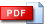# MATH103- Mathematics for Business and Ecconomics - I

Course Outline | Announcements |

### The Aim of the Course

Linear equations. Radical equations. Rational equations. Absolute value equalities. Quadratic equations. Linear inequalities. Absolute value inequalities. Quadratic inequalities. Rational inequalities. Rectangular coordinates. Linear functions. Lines. Paralel lines, Perpendicular lines.  Applications of linear functions; revenue, cost, profit applications, break – even point and equilibrium point. Linear inequalities. Applications of linear inequalities. Multiproduct Analysis. Systems of linear equations; 2x2, 3x3, 2x3 and 3x2 systems. Unique solution, infinitly many solution and no solution cases. Applications to the systems of linear equations. Quadratic functions. Parabolas. Applications of quadratic functions; revenue, cost, profit applications. Compound interest. Present value. Systems of linear inequalities. Linear Programming. Corner point method. LP Applications. Vectors. Matrices. Matrix operations. Transpose of a matrix. Determinants; determinants of 2x2 and 3x3 matrices.  Applications of matrices.

### Instructors

Dr. F. B. Rizaner (01,06), Dr. Şerife Bekar (02,03), Dr. H. Kaffaoğlu (04),Asst. Prof. N. Şemi (05)

### Tutorials

 Tutorial 1 - EquationsTutorial 2 - InequalitiesTutorial 3 - Sysytems and Applications of the systemsTutorial 4 - Linear Functions with applicationsTutorial 5 - Linear Programming with LP applicationsTutorial 6 - Quadratic Functions with applicationsTutorial 7 - Mathematics of Finance Tutorial 8- Matrix Algebra### Lecture Notes

 The EquationsThe InequalitiesThe systems with applicationsLinear FunctionsApplications of linear functions and inequalitiesLinear ProgrammingQuadratic Functions with applicationsMathematical FinanceMatrix Algebra### Sample Exam & Quiz Questions and Solutions

 Midterm1 Exam, Fall 2018-19Midterm1 Exam, Spring  2018-19